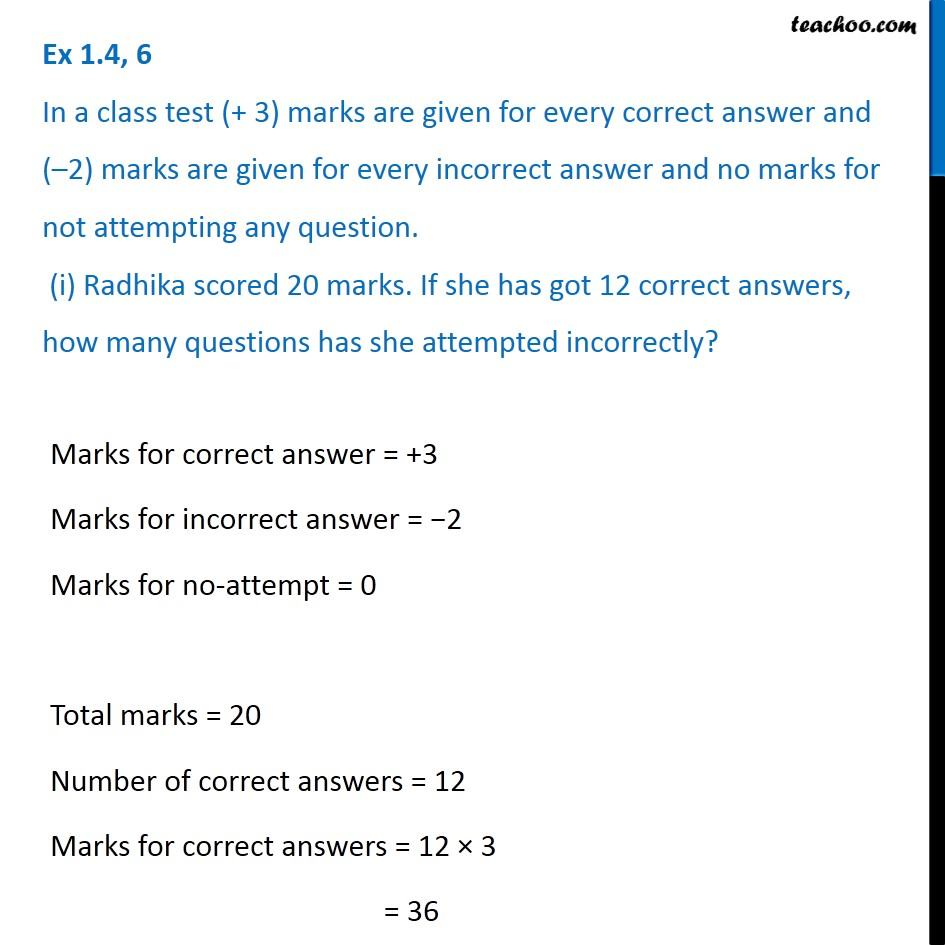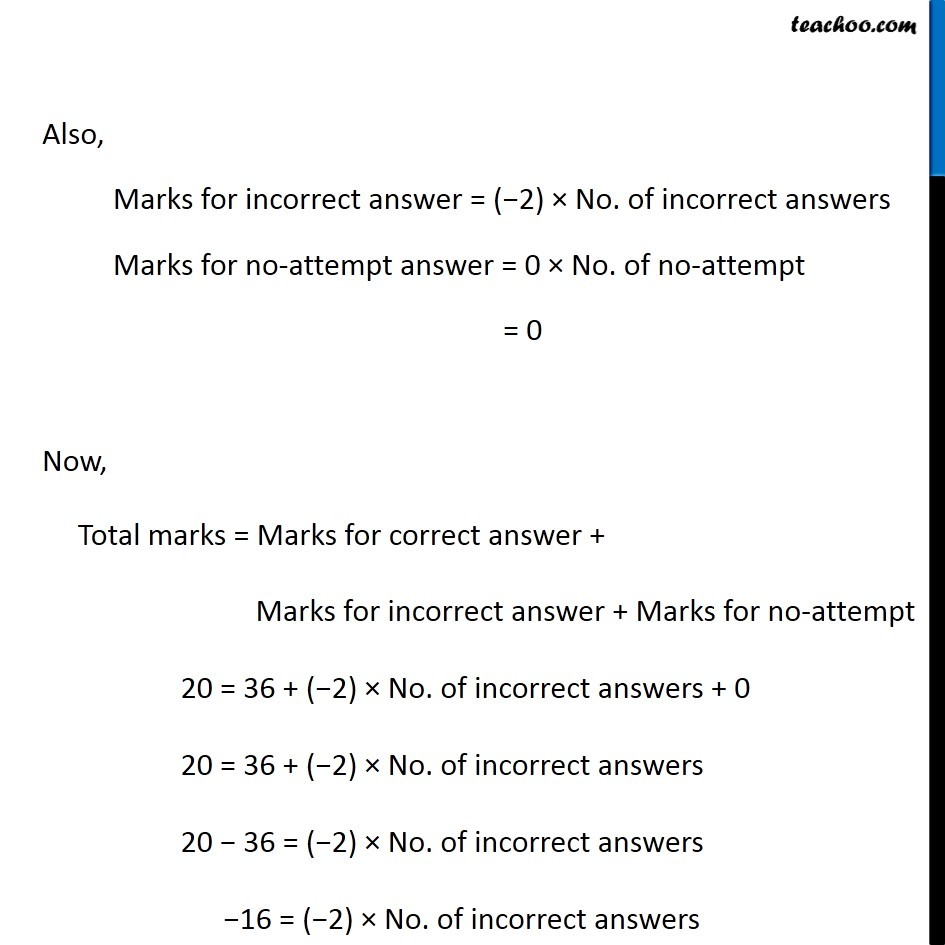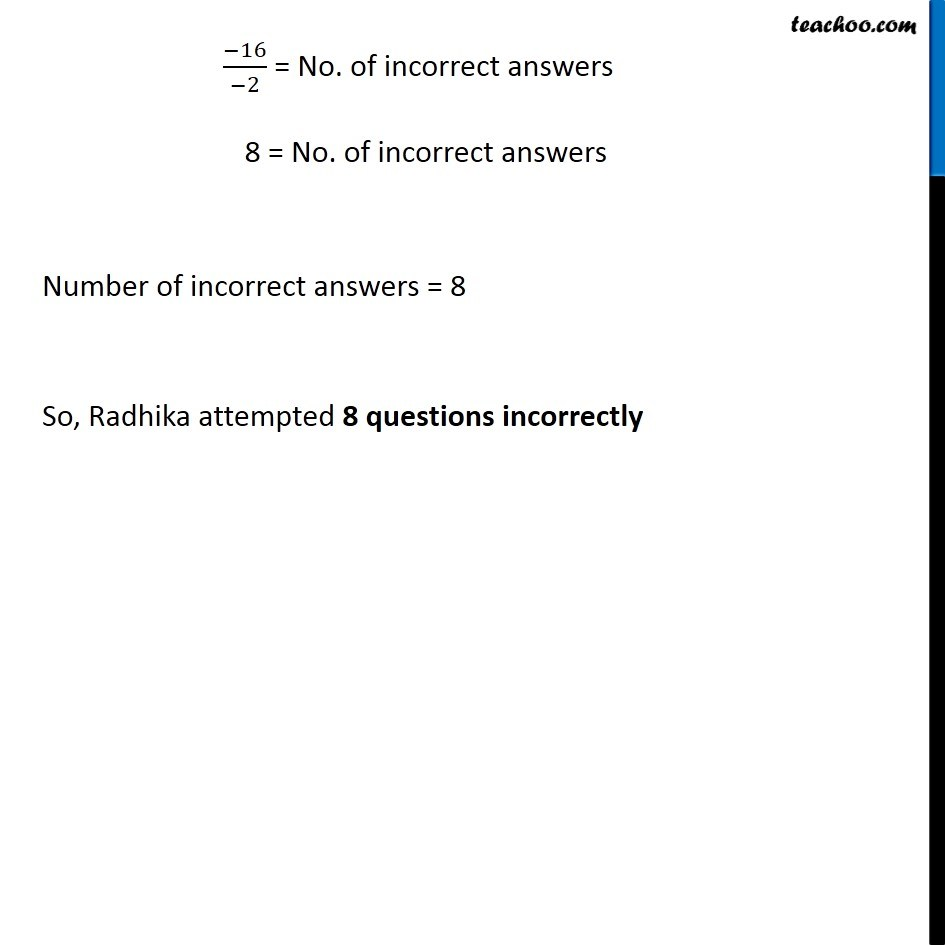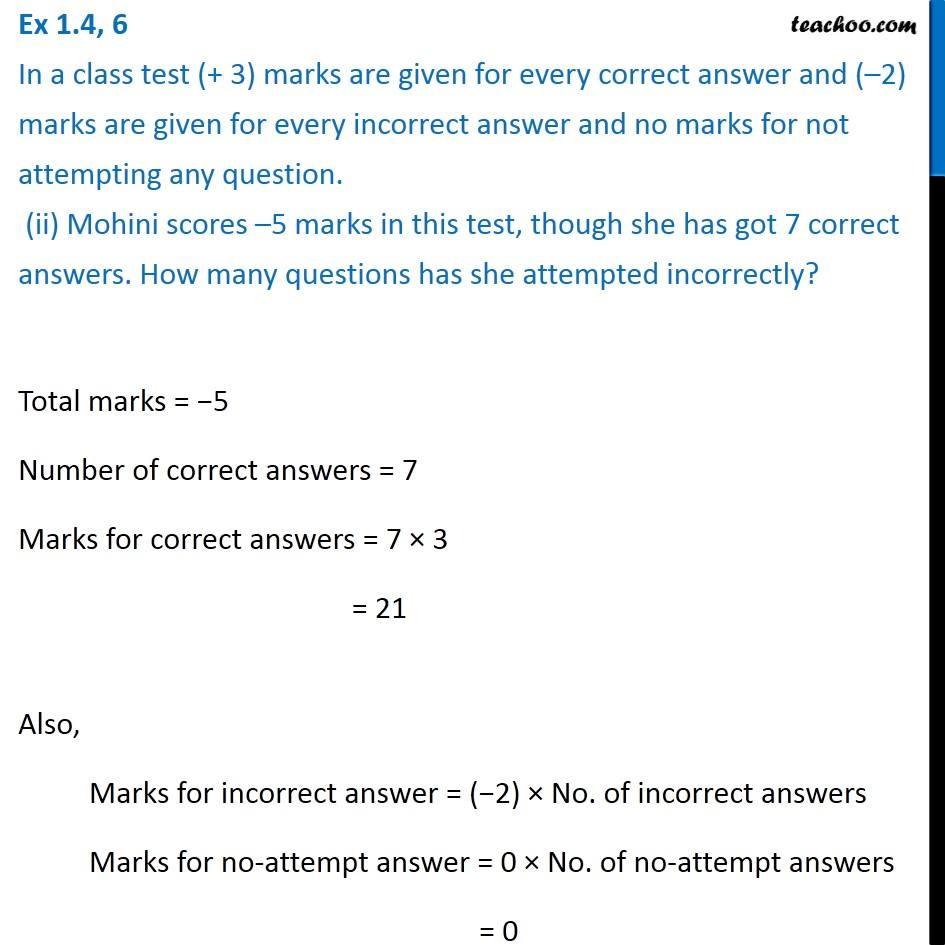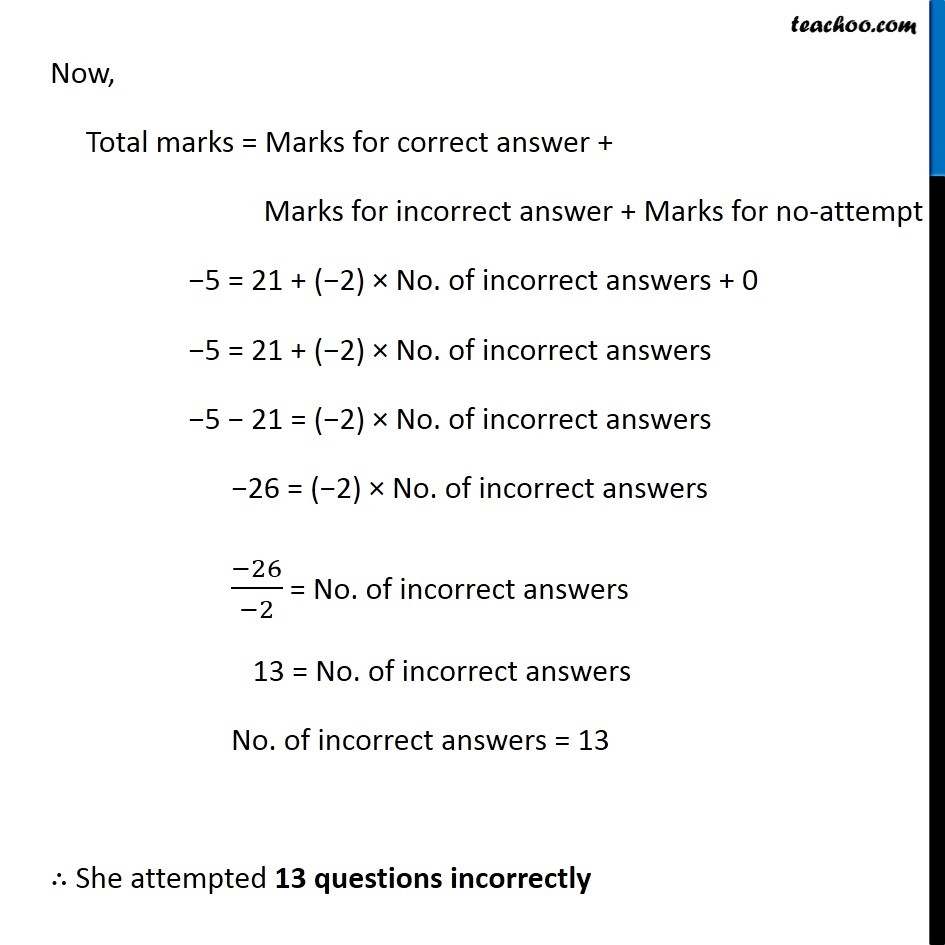1. Chapter 1 Class 7 Integers
2. Serial order wise
3. Ex 1.4

Transcript

Ex 1.4, 6 In a class test (+ 3) marks are given for every correct answer and ( 2) marks are given for every incorrect answer and no marks for not attempting any question. (i) Radhika scored 20 marks. If she has got 12 correct answers, how many questions has she attempted incorrectly? Marks for correct answer = +3 Marks for incorrect answer = 2 Marks for no-attempt = 0 Total marks = 20 Number of correct answers = 12 Marks for correct answers = 12 3 = 36 Also, Marks for incorrect answer = ( 2) No. of incorrect answers Marks for no-attempt answer = 0 No. of no-attempt = 0 Now, Total marks = Marks for correct answer + Marks for incorrect answer + Marks for no-attempt 20 = 36 + ( 2) No. of incorrect answers + 0 20 = 36 + ( 2) No. of incorrect answers 20 36 = ( 2) No. of incorrect answers 16 = ( 2) No. of incorrect answers ( 16)/( 2) = No. of incorrect answers 8 = No. of incorrect answers Number of incorrect answers = 8 So, Radhika attempted 8 questions incorrectly Ex 1.4, 6 In a class test (+ 3) marks are given for every correct answer and ( 2) marks are given for every incorrect answer and no marks for not attempting any question. (ii) Mohini scores 5 marks in this test, though she has got 7 correct answers. How many questions has she attempted incorrectly? Total marks = 5 Number of correct answers = 7 Marks for correct answers = 7 3 = 21 Also, Marks for incorrect answer = ( 2) No. of incorrect answers Marks for no-attempt answer = 0 No. of no-attempt answers = 0 Now, Total marks = Marks for correct answer + Marks for incorrect answer + Marks for no-attempt 5 = 21 + ( 2) No. of incorrect answers + 0 5 = 21 + ( 2) No. of incorrect answers 5 21 = ( 2) No. of incorrect answers 26 = ( 2) No. of incorrect answers ( 26)/( 2) = No. of incorrect answers 13 = No. of incorrect answers No. of incorrect answers = 13 She attempted 13 questions incorrectly

Ex 1.4

Chapter 1 Class 7 Integers
Serial order wise

About the AuthorDavneet Singh
Davneet Singh is a graduate from Indian Institute of Technology, Kanpur. He has been teaching from the past 9 years. He provides courses for Maths and Science at Teachoo.